## 1. Overview

In this tutorial, we’ll talk about the hidden layers in a neural network. First, we’ll present the different types of layers and then we’ll discuss the importance of hidden layers along with two examples.

## 2. Types of Layers

Over the past few years, neural network architectures have revolutionized many aspects of our life with applications ranging from self-driving cars to predicting deadly diseases. Generally, every neural network consists of vertically stacked components that are called layers. There are three types of layers:

• An Input Layer that takes as input the raw data and passes them to the rest of the network.
• One or more Hidden Layers that are intermediate layers between the input and output layer and process the data by applying complex non-linear functions to them. These layers are the key component that enables a neural network to learn complex tasks and achieve excellent performance.
• An Output Layer that takes as input the processed data and produces the final results.

Below we can see a simple feedforward neural network with two hidden layers: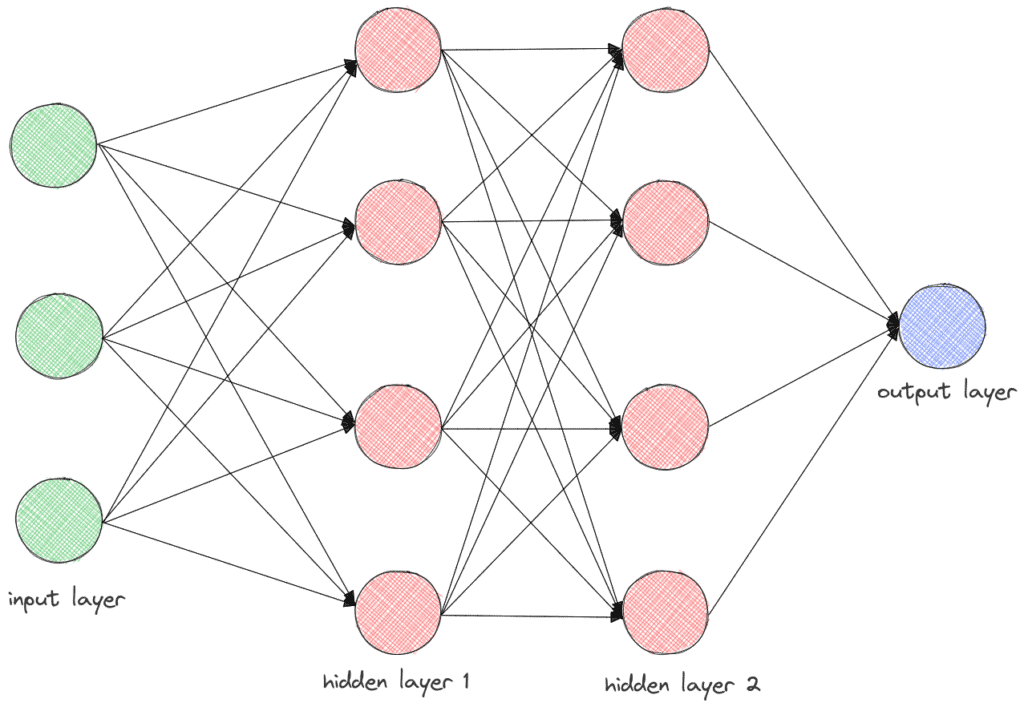In the above neural network, each neuron of the first hidden layer takes as input the three input values and computes its output as follows:whereare the input values,the weights,the bias andan activation function. Then, the neurons of the second hidden layer will take as input the outputs of the neurons of the first hidden layer and so on.

## 3. Importance of Hidden Layers

Now let’s discuss the importance of hidden layers in neural networks. As mentioned earlier, hidden layers are the reason why neural networks are able to capture very complex relationships and achieve exciting performance in many tasks.

To better understand this concept, we should first examine a neural network without any hidden layer like the one below that has 3 input features and 1 output: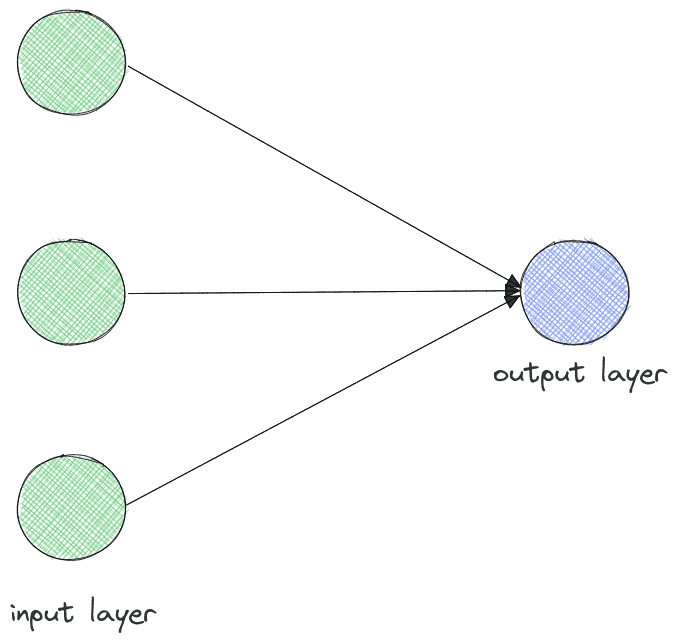Based on the previous equation, the output valueis equal to a linear combination along with a non-linearity. Therefore, the model is similar to a linear regression model. As we already know, a linear regression attempts to fit a linear equation to the observed data.

In most machine learning tasks, a linear relationship is not enough to capture the complexity of the task and the linear regression model fails. Here comes the importance of the hidden layers that enables the neural network to learn very complex non-linear functions.

## 4. Examples

Next, we’ll discuss two examples that illustrate the importance of hidden layers in training a neural network for a given task.

### 4.1. Logical Functions

Let’s imagine that we want to use a neural network to predict the output of an XOR logical gate given two binary inputs. According to the truth table ofXOR, the output is true whenever the inputs differ: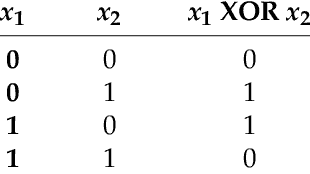To better understand our classification task, below we plot the four possible outputs. The green points correspond to an output equal to 1 while the red points are the zero outputs: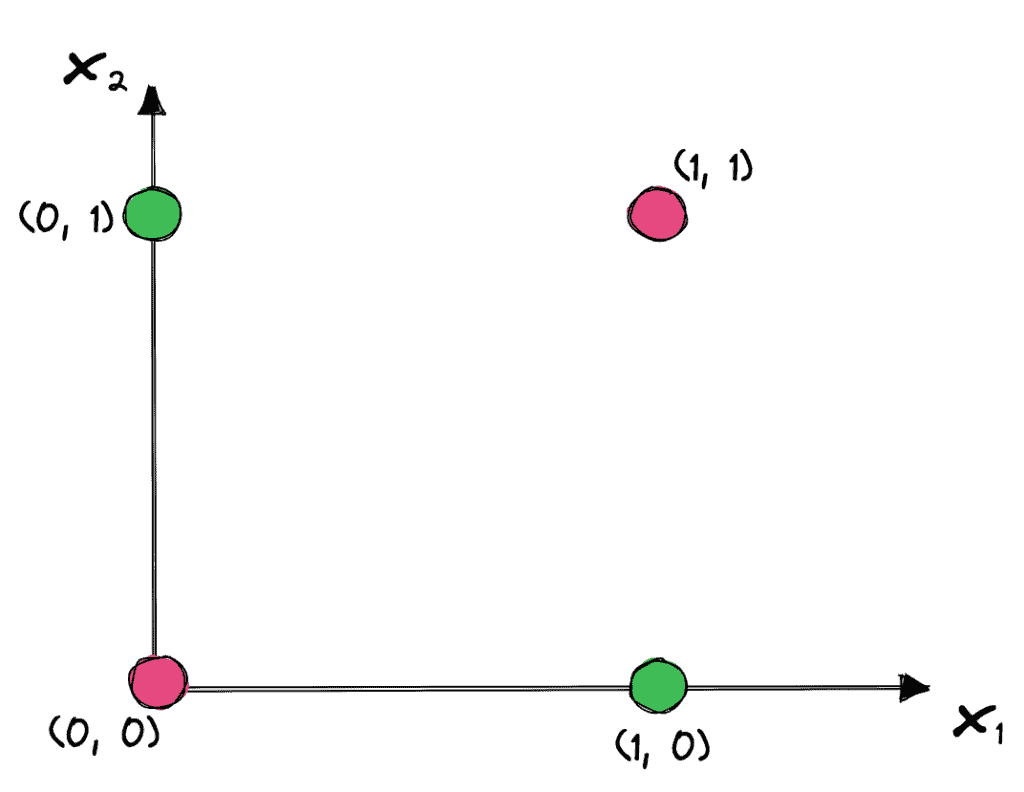At first, the problem seems pretty easy. The first approach could be to use a neural network without any hidden layer. However, this linear architecture is able to separate our input data points using a single line. As we can see in the above graph, the two classes cannot be separated using a single line and the XOR problem is not linearly separable.

Below, we can see some lines that a simple linear model may learn to solve the XOR problem. We observe that in both cases there is an input that is misclassified: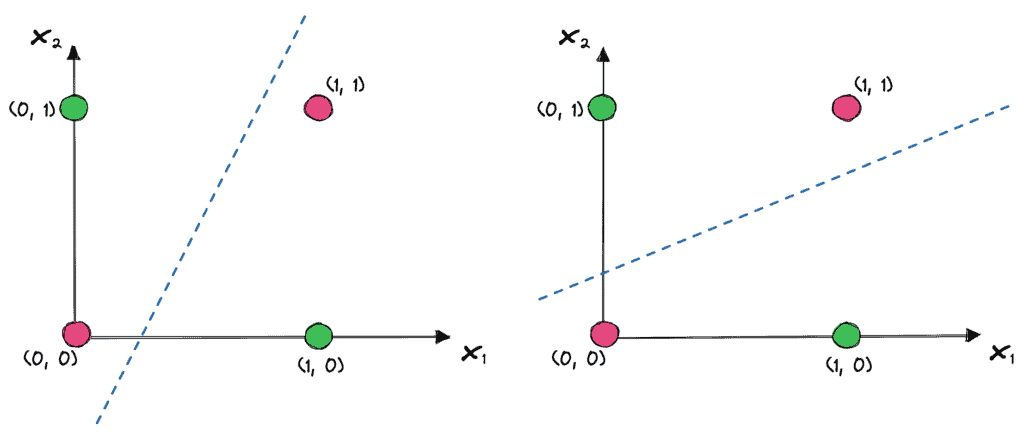The solution to this problem is to learn a non-linear function by adding a hidden layer with two neurons to our neural network. So, the final decision is made based on the outputs of these two neurons that each one learns a linear function like the ones below: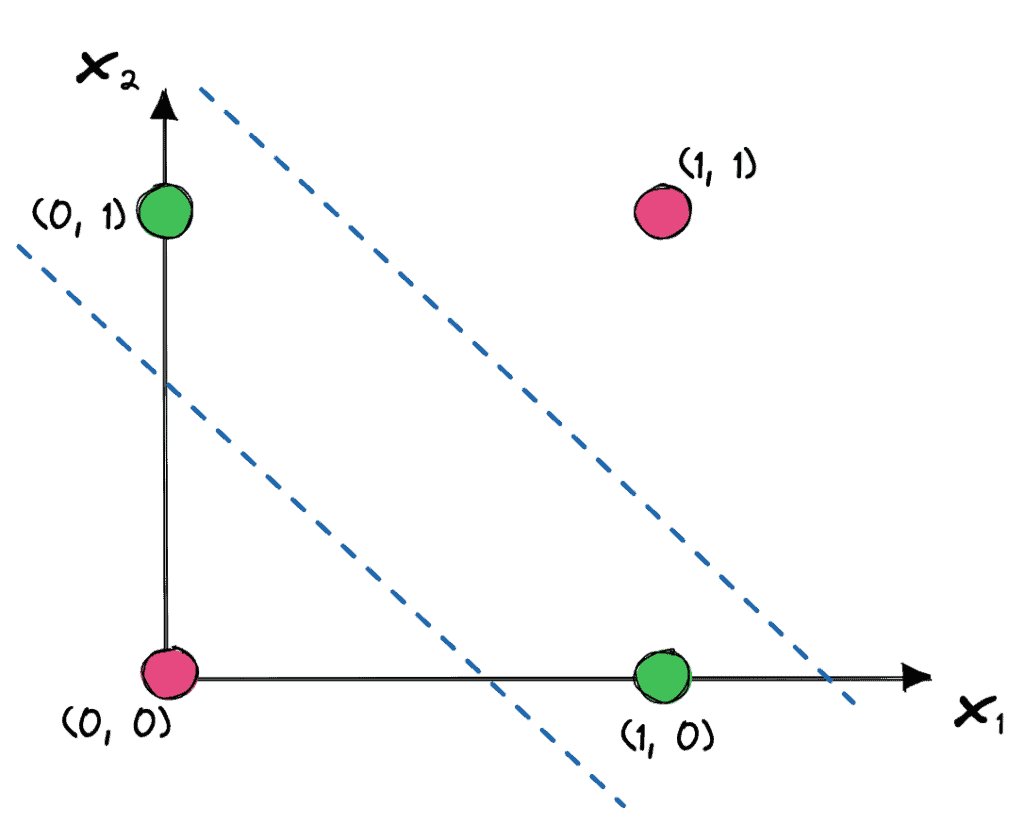The one line makes sure that at least one feature of the input is true and the other line makes sure that not all features are true. So, the hidden layer manages to transform the input features into processed features that can then be correctly classified in the output layer.

### 4.2. Images

Another way to realize the importance of hidden layers is to look into the computer vision domain. Deep neural networks that consist of many hidden layers have achieved impressive results in face recognition by learning features in a hierarchical way.

Specifically, the first hidden layers of a neural network learn to detect short pieces of corners and edges in the image. These features are easy to detect given the raw image but are not very useful by themselves to recognize the identity of the person in the image. Then, the middle hidden layers combine the detected edges from the previous layers to detect parts of the face like the eyes, the nose and the ears. Then, the final layers combine the detectors of the nose, eyes, etc to detect the overall face of the person.

In the image below, we can see how each layer helps us to go from the raw pixels to our final goal: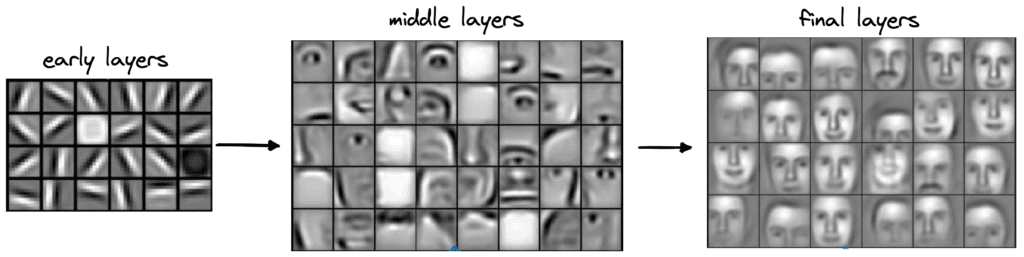## 5. Conclusion

In this tutorial, we talked about the hidden layers in a neural network. First, we discussed the types of layers that are present in a neural network. Then, we talked about the role of hidden layers and provided two examples to better understand their importance.